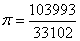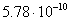Pi (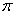) is a universal constant indicate the ratio of circumference of a circle and its diameter. The value of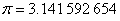has been estimated since Archimedes (see: Phillips, 2000 ).

Using MS excel, we can use function =PI() to represent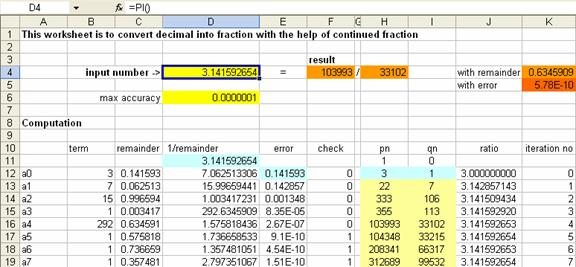## Example

 Accuracy Pi as ratio Error (computed from PI of MS Excel) 0.1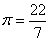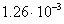0.001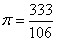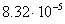0.00001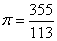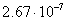0.0000001